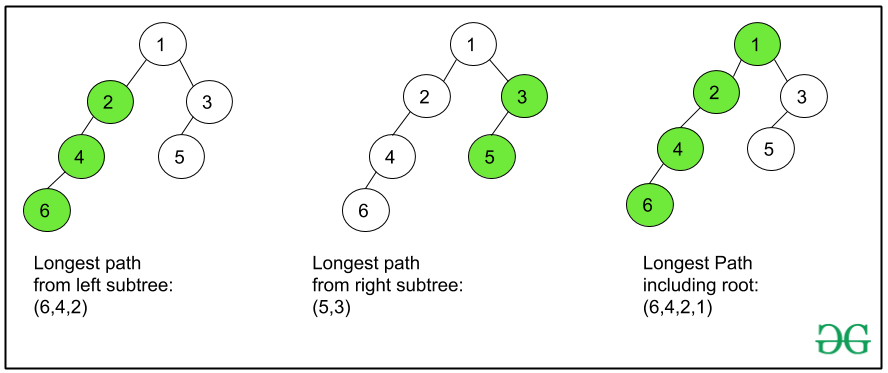# Print the longest path from root to leaf in a Binary tree

Given a binary tree, the task is to print the longest path from the root node to the leaf node. If there are multiple answers print any one of them.
Examples:

```Input:
4
/ \
3   6
/ \
5   7
Output:
4 -> 6 -> 7
Explanation:
Longest paths from root to leaf
are (4 -> 6 -> 5)
and (4 -> 6 -> 7).
Print any of them.

Input:
1
/ \
2   3
/  \
4    5
\
6
Output:
1 -> 2 -> 5 -> 6
```

Naive Approach: The idea is to generate all possible paths from the root node to all leaf nodes, keep track of the path with maximum length, finally print the longest path.
Time Complexity: O(N2)

Efficient Approach: The idea is to use Recursion to solve this problem efficiently. The main idea is to recursively get the longest path from left subtree and right subtree then add the current node to one which has a greater length and it will be the longest path from the current node to leaf. Starting with the root node, follow the steps below for each node called recursively.

• If the root node is null then no path exists, return an empty vector.
• Get longest path from right subtree in a vector rightvect by recursively traversing root -> right.
• Similarily, get longest path from left subtree in a vector leftvect by recursively traversing root -> left.
• Compare the length of rightvect and leftvect and append the current node to the longer of the two and return that vector.

By following the above steps, the vector obtained at the end of the tree traversal, is the longest path possible. Print the vector in reverse as the longest path from root to leaf.

Look at this image to understand how the longest paths from the nodes of left and right subtree are used to obtain the longest path from the current node:Below is the implementation of the above approach:

## C++

 `// C++ Program to print Longest Path ` `// from root to leaf in a Binary tree ` `#include ` `using` `namespace` `std; ` ` `  `// Tree node Structure ` `struct` `Node { ` `    ``int` `data; ` `    ``Node *left, *right; ` `}; ` ` `  `struct` `Node* newNode(``int` `data) ` `{ ` `    ``struct` `Node* node = ``new` `Node; ` `    ``node->data = data; ` `    ``node->left = node->right = NULL; ` ` `  `    ``return` `(node); ` `} ` ` `  `// Function to find and return the ` `// longest path ` `vector<``int``> longestPath(Node* root) ` `{ ` ` `  `    ``// If root is null means there ` `    ``// is no binary tree so ` `    ``// return a empty vector ` `    ``if` `(root == NULL) { ` `        ``vector<``int``> temp ` `            ``= {}; ` `        ``return` `temp; ` `    ``} ` ` `  `    ``// Recursive call on root->right ` `    ``vector<``int``> rightvect ` `        ``= longestPath(root->right); ` ` `  `    ``// Recursive call on root->left ` `    ``vector<``int``> leftvect ` `        ``= longestPath(root->left); ` ` `  `    ``// Compare the size of the two vectors ` `    ``// and insert current node accordingly ` `    ``if` `(leftvect.size() > rightvect.size()) ` `        ``leftvect.push_back(root->data); ` ` `  `    ``else` `        ``rightvect.push_back(root->data); ` ` `  `    ``// Return the appropriate vector ` `    ``return` `(leftvect.size() > rightvect.size() ` `                ``? leftvect ` `                ``: rightvect); ` `} ` ` `  `// Driver Code ` `int` `main() ` `{ ` `    ``struct` `Node* root = newNode(1); ` `    ``root->left = newNode(2); ` `    ``root->right = newNode(3); ` `    ``root->left->left = newNode(4); ` `    ``root->left->right = newNode(5); ` `    ``root->left->right->right = newNode(6); ` ` `  `    ``vector<``int``> output = longestPath(root); ` `    ``int` `n = output.size(); ` ` `  `    ``cout << output[n - 1]; ` `    ``for` `(``int` `i = n - 2; i >= 0; i--) { ` `        ``cout << ``" -> "` `<< output[i]; ` `    ``} ` ` `  `    ``return` `0; ` `} `

Output:

```1 -> 2 -> 5 -> 6
```

Time complexity: O(N)
Auxiliary Space: O(N)

Attention reader! Don’t stop learning now. Get hold of all the important DSA concepts with the DSA Self Paced Course at a student-friendly price and become industry ready.

My Personal Notes arrow_drop_upCheck out this Author's contributed articles.

If you like GeeksforGeeks and would like to contribute, you can also write an article using contribute.geeksforgeeks.org or mail your article to contribute@geeksforgeeks.org. See your article appearing on the GeeksforGeeks main page and help other Geeks.

Please Improve this article if you find anything incorrect by clicking on the "Improve Article" button below.